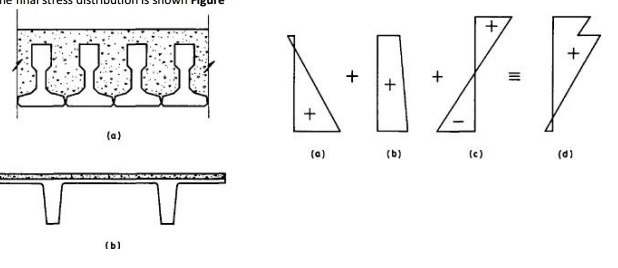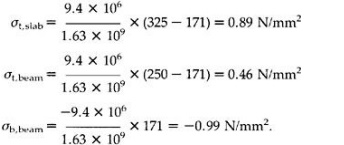Home | | Prestressed Concrete Structures | Concrete Structures: Serviceability Limit State

# Concrete Structures: Serviceability Limit StateOnce the concrete in the slab has hardened and the imposed load acts on the composite section, the additional stress distribution.

SERVICEABILITY LIMIT STATE

The stress distributions in the various regions of the composite member are shown in Fig. 10.2(a)-(d). The stress distribution in Fig. 10.2(a) is due to the self weight of the beam, with the maximum compressive stress at the lower extreme fibre. Once the slab is in place, the stress distribution in the beam is modified to that shown in Fig. 10.2(b), where the bending moment at the section, Md is that due to the combined self weight of the beam and slab.

Once the concrete in the slab has hardened and the imposed load acts on the composite section, the additional stress distribution is shown in Fig. 10.2(c). This is determined by ordinary bending theory, but using the composite section properties.

The final stress distribution is shown FigureStress distribution within a composite section. The floor slab shown in Fig. 10.3 comprises precast pretensioned beams and an in situ concrete slab. If the span of the beams is 5 m and the imposed load is 5 kN/m2 (including finishes), determine the stressdistributions at the various load stages. Assume all long-term losses have occurred before the beams are erected and that the net force in each wire is 19.4 kN. Section properties of the beams:

Ac=1.13�105 mm2

Ic=7.5�108 mm4

Zt=Zb=6�106 mm3.

Eccentricity of the wires=125?40=85 mm.

(i)                                                                                                                                                                                                       Self weight of the beams=0.113�24

=2.7 kN/m.

Mo=(2.7�52)/8

=8.4 kNm.

Total prestress force after all losses have occurred is given by

�Po=6�19.4

=116.4 kN.

The stress distribution in the beams is thus given by(ii)                                                                                                                                                                                                     The weight of the slab is supported by the beams acting alone, so that d=8.4+0.075�0.6�24�52/8 =11.8 kNm. The stress distribution within the beams is now given

(iii)                                                                                                                                                                                                   (iii) The imposed load of 5 kN/m2 is supported by the composite section and the section properties of this are now required. To find the neutral axis of the composite section, taking moments about the soffit of the beams gives

(1.13�105+75�600)y=(1.13�105�125+75�600�288)

?y=171 mm.

Icomp=7.5�108+1.13�105 (171-125)2+(753�600)/12+(75�600)/(288-171)2

=1.63�109 mm4.

The imposed load bending moment, (Mdes?Md)=0.6�5�52/8

=9.4 kNm. The stress distribution within the composite section under this extra bending moment

is given byThe maximum compressive stress occurs at the upper fibres of the beams, but is significantly lower than the level of stress had the beam carried the total imposed load alone. This explains the advantage of inverted T-sections in composite construction, where only a small compression flange is required for bending moments Mo and Md, theStress distribution for composite section in Example 10.1 (N/mm2): (a) beam; (b)beam and slab; (c) beam and slab and imposed load.

compression flange for bending moment Mdes being provided by the slab. The maximum compressive stress in the slab is much lower than in the beam and, for this reason, in many composite structures a lower grade of concrete is used for the in situ portion. The modulus of elasticity for this concrete is lower than that for the beam and this effect can be taken into account in finding the composite section properties by using an approximate modular ratio of 0.8.

The in situ slab in Example 10.1 lies above the composite section neutral axis and, therefore, the slab is in compression over its full depth under the total design load. However, for composite sections as shown in Fig. 10.1(a) the in situ portion of the section extends well below the neutral axis, so that the lower region is in tension. If the tensile strength of this concrete is exceeded then the composite section properties must be determined on the basis of the in situ section having cracked below the neutral axis.

Study Material, Lecturing Notes, Assignment, Reference, Wiki description explanation, brief detail
Civil : Prestressed Concrete Structures : composite construction : Concrete Structures: Serviceability Limit State |

Related Topics